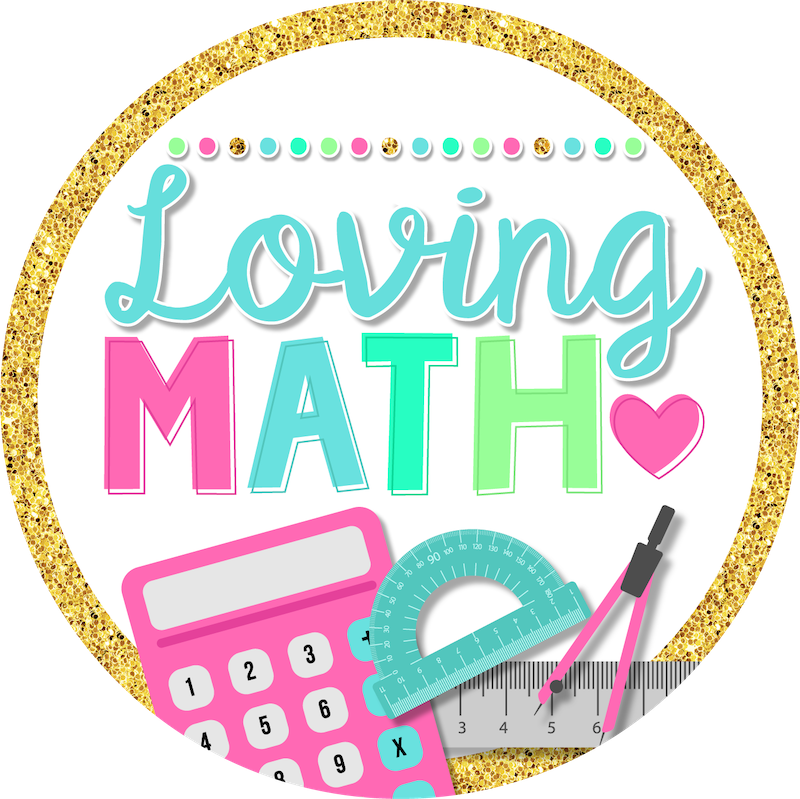# Effective Ways to Teach Word Problems

Word problems can be tricky for a lot of students, but they’re incredibly important to master.

For many students who struggle with mathematics, word problems are just a jumble of words and numbers. However, you can help students make sense of these problems by teaching them problem-solving processes. Indeed, as students move forward in their mathematical learning, they will need to apply problem-solving processes to more and more complex situations so they become successful problem solvers.

### Strategies to Teach Word Problems

To solve a word problem, students need to understand its context and develop a strategy to solve it.

One strategy is to use a process chart, which can guide students as they tackle a new problem. It helps to focus on how each step of the process supports students as they work to access the problem. An example problem-solving process is provided below:

• Read the problem, then reread it and highlight key words and numbers. Reading the problem a second time with annotations helps students sort out the core information from the background noise.
• Draw a picture of the situation that the problem presents. It may be helpful to first visualize a story or imagine a movie scene. Visualizing a story can be a powerful strategy that helps students create a picture or diagram of the problem.
• Determine the goal of the problem.
• Establish a strategy or write an equation to represent the picture. Estimate an answer, if possible. Estimating or approximating an answer helps students decide if they are on the right track.
• Solve the problem and check the reasonableness of your answer. Reminding students that it is rare to complete a problem correctly on the first attempt encourages them to embrace mistakes and errors

### Resources you can Use to Teach Word Problems

There are many resources that can support students as they work to solve these problems and expand the strategies they have learned in the classroom.

Using manipulatives, whether physical or virtual, is a great way for students to recreate the problem and be able to make sense of it.

Here is a great website that contains many virtual manipulatives for your students.

Bar models are also a great way to visually represent the problem. When using bar models students are much more likely to recognize which operation is needed to solve. Bar models provide a “guide” or stating point for students who get stuck and don’t know where to begin. As a result, they are more likely to be engaged with the problem at hand.

A bar model is a pictorial representation of a problem or concept where bars or boxes are used to represent the known and unknown quantities. Bar models are most often used to solve number problems with the four operations – addition and subtraction, multiplication and division.

Bar models help children decide which operations to use or visualize problems.

### Practice is key

A big reason why students struggle so much with this skill is lack of practice. Many math textbooks have a section for word problems at the end of the lesson or at the end of the chapter.

You might wonder….

What is the problem with that?

Well, since students know the word problems are connected to the skill being taught, they already know what operation is needed to solve before they even read the problem. It is pointless to use these problems since it defeats the purpose of problem solving.

Instead, use a variety of word problems in your classroom that are unrelated to the daily skills being taught. This should be done DAILY and strategies should be shared since each student might approach the problem a different way.

I have created a resource just for you!

One Each Day Word Problems was born out of the need for my students to have daily practice. There is a problem for each day and the skills progress each month as you teach your curriculum.

This resource is great for you if you teach 4th grade as it includes most of the skills taught in this grade level.

Get One Each Day for each month of the year and get your students fluent in problem solving!

August Word Problems

September Word Problems

October Word Problems

November Word Problems

CUBES Strategy Word Problems